Latest Banking jobs   »

# Quantitative Aptitude Quiz For IDBI AM/Executive 2022- 5th July

Directions (1-5): In each of these questions, two equations (I) and (II) are given. You have to solve both the equations and give answer
(a) if x > y                                                                        .
(b) if x ≥ y                                                                           .
(c) if x < y
(d) if x ≤ y                                                                                          .
(e) if x = y or no relation can be established between x and y.

Quantitative Quiz For IDBI AM/Executive 2022 5th July CHECK IN HINDI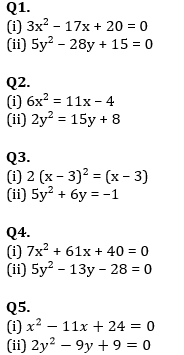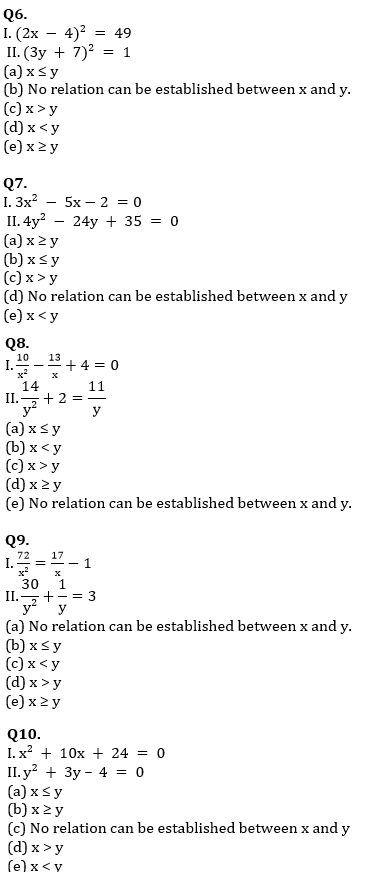Directions (11-15): In the following questions, two equations numbered I and II are given. You have to solve both the equations and give answers among the following options.                                               .
(a) if x > y
(b) if x ≥ y
(c) if x < y
(d) if x ≤ y
(e) if x = y or the relationship cannot be established.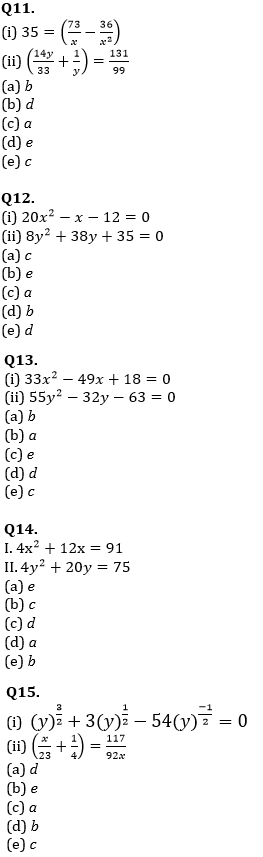Solutions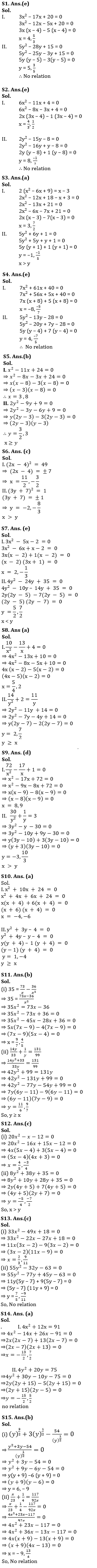#### Congratulations!Incorrect details? Fill the form again here

•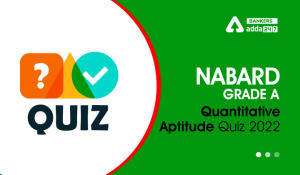Quantitative Aptitude Quiz For NABARD Gr...
•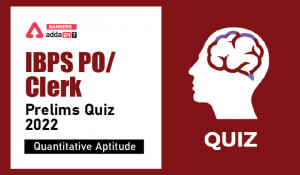Quantitative Aptitude Quiz For IBPS Cler...
•Quantitative Aptitude Quiz For IBPS RRB ...
•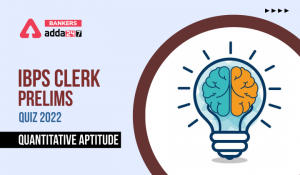Quantitative Aptitude Quiz For IBPS Cler...
•Quantitative Aptitude Quiz For IBPS RRB ...
•Quantitative Aptitude Quiz For IBPS Cler...